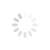# How can students deal with fear of mathematics subject?

Written By: / Friday, 02 April 2021 17:09

The common problem faced by students is, solving the difficult questions and doing quick calculations which can only be possible with regular practice. Moreover, if a student is already getting bad grades in maths then their fear of failing the subject comes to its peak.
Every student who has to appear for mathematics exam gathers the courage to face the tough questions. The common problems faced by the students for mathematics are discussed here –
Common problems of students for mathematics paper –
They go blank and confused on seeing mathematics question paper
They make minor mistakes while solving the questions
Difference between weak and brilliant students –
A student who is brilliant in mathematics gets to know about the type of questions and prepares a strategy accordingly by just solving one or two questions from a chapter. And students who are weak in mathematics get confused and, soon give up solving the questions halfway through.
Weak students need to refer the basic concept for each and every question. However, students, who are quick in mathematics, pick the questions quickly after understanding the concept at once only.
How to deal with maths like toppers
Do more practice– Mathematics is the only subject where learning theory is not so important as solving the verity of numerical So, the students who are weak in mathematics need to solve as many questions as possible to increase their speed and accuracy in mathematics.
Use mental calculations – The students should use mental calculations or aptitude to solve basic parts of the questions. It is commonly observed that students get stuck with complex calculations but with the mental calculations of even simple arithmetic, they will be able to save their extra time and efforts. Topper students accept this fact that for mathematics subject they do mental calculations to play with numbers and get quick and accurate.
Divide the concepts – Students who are weak in mathematics should take small steps to master maths. For this, they need to divide the questions from a concept into three parts –
Easy –questions directly based on the theorems and formulae.
Moderate – The questions based on 2 or more concepts.
Tough questions – The lengthy and time taking questions which need to be solved with different strategies; for example questions in probability chapters.
Don’t adopt new tricks for solving questions – Students need to understand that maths is the only subject where changing methods and techniques of learning to solve the questions are rare. We have been adapting to the methods which the math experts have taught us over the decades. However, there are interesting and fun tricks to learn mathematics such as Vedic mathematics, but if students who are not well aware of it then they should not try it during their exams. Such methods of learning Maths should be practice when students have enough time to spare and they want to learn something new and interesting.
Conclusion: Students, who fear mathematics but have to appear for the subject in examinations, need not worry rather they should develop a plan and strategy to overcome this challenge. Mathematics is a subject where students can get marks easily if they implement correct formula and method. Use the tips mentioned here to do away your maths fear and get good marks on the subject.

### About the Author### Vijay Garg

Vijay Garg is a regular contributor of Imphal Times, mostly related with Education. Vijay is a resident of Street Kour Chand MHR Malout-152107 Distt Sri Muktsar sahib Punjab. Vijay Garg, Ex.PES-1 is a retired Principal from Government Girls Sen Sec school Mandi Harji Ram Malout -152106 Punjab. He is also the author of Quantitative Aptitude, NTSE , NMMS, Mathematics of XII, ICSE numerical physics and chemistry many more books.

Please publish modules in offcanvas position.